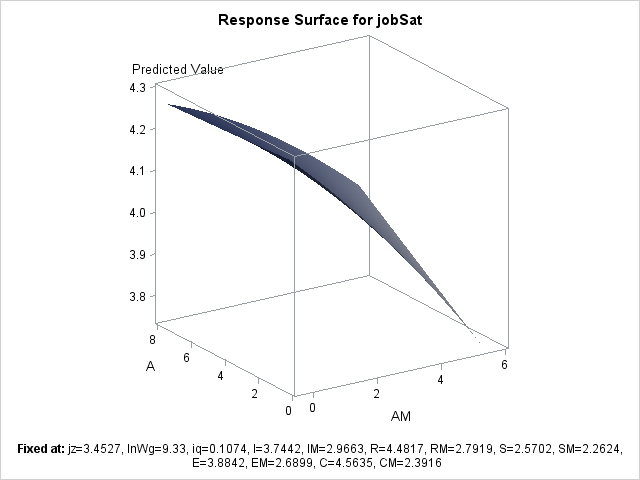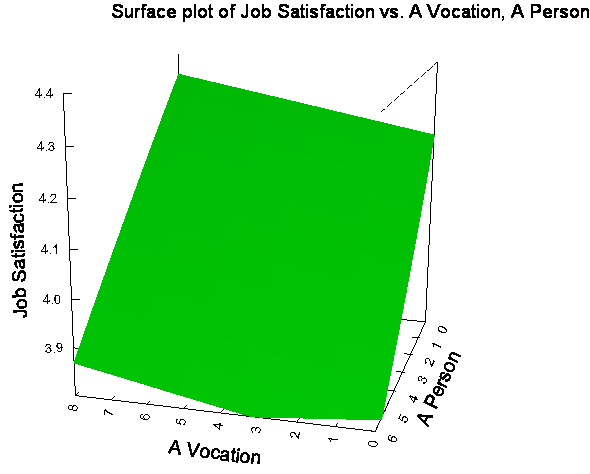## PROC RSREG [alternative procedure may be required]: plot response surface using ONLY linear terms

Hello all,

Please see my example syntax below.  In the case below, my quadratic and interaction terms are not signficant, but my linear terms are (for A and AM, the two variables compared on the response surface).  As such, I am interpretting only the linear terms.  I would like to plot a response surface using only the linear terms (i.e. an entirely flat 3-D surface).  I can do this in other software using a 3D function plot.  Is there a way to do this within PROC RSREG?  If not, what is the best way to plot the linear 3-D response surface in SAS?

Many thanks!

``````ods graphics on;
title "M & Job Sat & A";
proc rsreg data=pt8
plots=(surface(3d rotate=-33 fill=pred));
model jobSat= jz lnWg iq I IM R RM S SM E EM C CM A AM /lackfit /covar=13 ; where sex=0;
run;
ods graphics off;
``````
2 REPLIES 2

## Re: PROC RSREG [alternative procedure may be required]: plot response surface using ONLY linear ter

By experimentation, it looks like RSREG needs at least two quadratic terms to plot a surface. It looks like you have 15 covariates, and you are using COVAR=13 to set the first 13 to linear. Therefore I would have guessed that the surface would appear.

Then use the OUT= and PREDICT options to write the predicted values to a SAS data set on a uniform grid of points.You can then construct a surface plot by following the steps on my blog.

If you want to visualize linear surface (or even a quadratic on), I recommend using a contour plot instead. It will be much easier to estimate the height of the surface if you can see the contours .

## Re: PROC RSREG [alternative procedure may be required]: plot response surface using ONLY linear ter

Hi @Rick_SAS

You need at least two variables not set as covariates to get a plot (i.e. in my case, setting covariates to 15 will not plot anything at all).  Unfortunately, rsreg ONLY produces a quadratic plot(s) with the two (or more) variables you don't set as covariates (the final variables in the list)--it will not plot a surface using the linear results of the two terms as I desire.

To help visualize, I've attached the resreg quadratic output ("rsreg.png") and the corresponding linear plot (using SYSTAT; "systat.png").  I'd like to be able to make the linear plot in SAS as well.  Additional thoughts on how to accomplish this?Discussion stats
• 2 replies
• 783 views
• 0 likes
• 2 in conversation